Evaluate

Evaluate is used to ensure that one or more argument of a function evaluates even if the argument(s) would have not evaluated due to one of the attributes HoldAll, HoldRest, HoldFirst.  As an example consider the definitions in the next cell.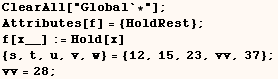Because  f  has the HoldRest attribute only the first argument of  f  evaluates before the definition of  f  is used.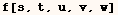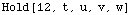In the next cell Evaluate is used to ensure theargument evaluates when it would have been held.  Notice (v) evaluated to (vv), and then (vv)  evaluated to 28.  It would be very difficult to make (v) evaluate to (vv), but prevent evaluation of (vv) to 28.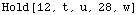We can give Evaluate multiple arguments and they evaluate to a sequence as in the next cell.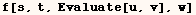You might expect the following to evaluate the (v) in {u,v}, but it doesn't work. That is because in order for Evaluate to have an effect, the expression of which it is an argument must be evaluated.  In this example Evaluate[v]  is an argument of {u,Evaluate[v]}, and this list doesn't evaluate because (f) has the HoldRest attribute.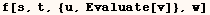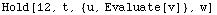In order to evaluate something deep inside a held expression you have to do something like the following.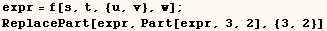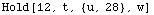Common applications for Evaluate

The first argument of  Plot[f, {x,xmin,xmax}]  must evaluate to a machine size real number when (x) is substituted fro a value between (xmin) and (xmax).  Plot also allows the form  Plot[{f1,f2,f3}, {x,xmin,xmax}] but this only works when the first argument of Plot has the head List before the Plot algorithm is called.  Sometimes we need to Evaluate the first argument of Plot to give it what it needs.  Several examples of this are given below.

In the first three examples Evaluate is needed to ensure the first argument has the head List.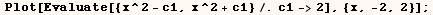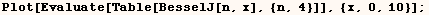If Evaluate isn't used in the next example the Plot algorithm tries to take the derivative with respect to numeric values such as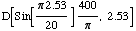but this is nonsense.  The solution is to use Evaluate to ensure the derivative is computed before the Plot algorithm is called.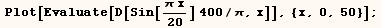Created by Mathematica  (May 16, 2004)

Back to Ted’s Tricks index page